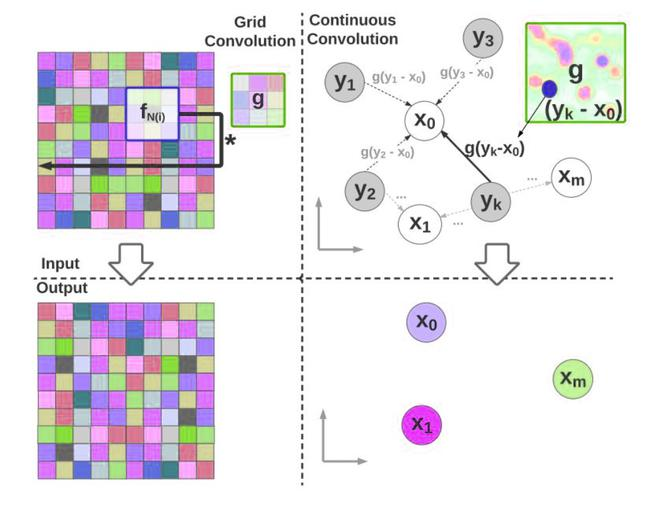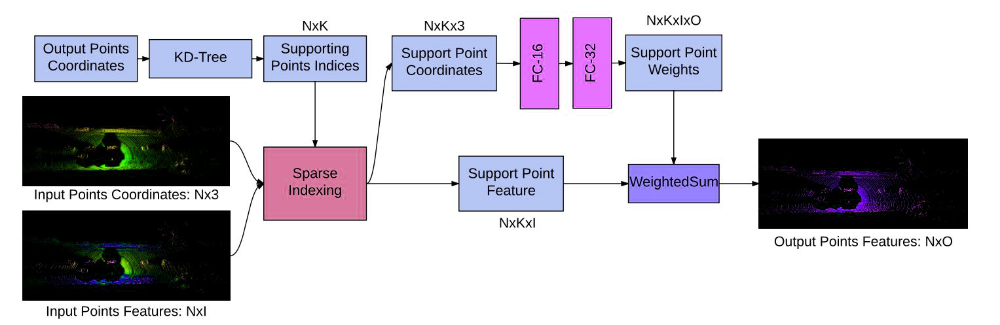# Deep parametric Continuous Convolutional Neural Network

• Last Updated : 15 Sep, 2021

Deep Parametric Continuous Kernel convolution was proposed by researchers at Uber Advanced Technologies Group. The motivation behind this paper is that the simple CNN architecture assumes a grid-like architecture and uses discrete convolution as its fundamental block. This inhibits their ability to perform accurate convolution to many real-world applications. Therefore, they propose a convolution method called Parametric Continuous Convolution.

#### Parametric Continuous Convolution:

Parametric Continuous Convolution is a learnable operator that operates over non-grid structured data and explores parameterized kernels that span over full continuous vector space. It can handle arbitrary data structures as far as the support structure is computable. The continuous convolution operator is approximated to a discrete  by Monte Carlo sampling:The next challenge is to define g, which is parameterized in such a way that each point in the support domain is assigned a value. This is impossible since it requires g to be defined over infinite points of a continuous domain.Grid vs Continuous conv

Instead, the authors use multi-layer perceptron as an approximate parametric continuous convolution function because they are expressive and able to approximate the continuous functions.The kernel g(z,∅ ): RD→ R spans over full continuous support domains while remaining parameterized by a finite number of computations

#### Parametric continuous Convolution Layer:

The Parametric continuous convolution layer has 3 parts:

• Input Feature Vector• Associated Location in Support domain• Output domain locationFor each layer, we first evaluate the kernel function:; given parameter. Each element of the output vector can be calculated as:where, N be the number of input points, M be the number of output points, and D the dimensionality of the support domain and F and O be predefined input and output feature dimensions respectively. Here, we can observe the following difference from discrete convolution:

• The kernel function is a continuous function given the relative position in the support domain.
• The (input, output) points could be any points in the continuous domain as well and can be different.

#### Architecture:

The network takes the input feature and their associated position in the support domain as input. Following standard CNN architecture, we can add batch normalization, non-linearities, and the residual connection between layers which was critical to helping convergence. Pooling can be employed over the support domain to aggregate information.Deep Para CKConv architecture

#### Locality Enforcing Convolution

The standard convolution computed over a limited kernel size M to enforce locality in the discrete scenarios.  However, the continuous function can enforce locality by computing the function that finds the points closer to x.Where, w() is a modulating window function to enforce locality. It uses the k-Nearest Neighbor in its algorithm.

#### Training

Since, all the building blocks of the model can be differentiable within their domain, so, we can write the backpropagation function as:#### References:

My Personal Notes arrow_drop_up